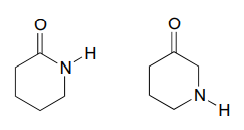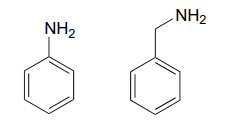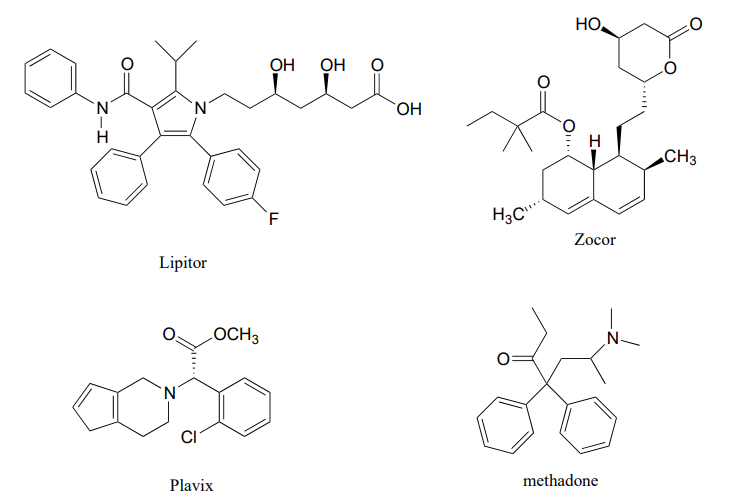# 7.E: Acid-base Reactions (Exercises)

$$\newcommand{\vecs}{\overset { \rightharpoonup} {\mathbf{#1}} }$$ $$\newcommand{\vecd}{\overset{-\!-\!\rightharpoonup}{\vphantom{a}\smash {#1}}}$$$$\newcommand{\id}{\mathrm{id}}$$ $$\newcommand{\Span}{\mathrm{span}}$$ $$\newcommand{\kernel}{\mathrm{null}\,}$$ $$\newcommand{\range}{\mathrm{range}\,}$$ $$\newcommand{\RealPart}{\mathrm{Re}}$$ $$\newcommand{\ImaginaryPart}{\mathrm{Im}}$$ $$\newcommand{\Argument}{\mathrm{Arg}}$$ $$\newcommand{\norm}{\| #1 \|}$$ $$\newcommand{\inner}{\langle #1, #2 \rangle}$$ $$\newcommand{\Span}{\mathrm{span}}$$ $$\newcommand{\id}{\mathrm{id}}$$ $$\newcommand{\Span}{\mathrm{span}}$$ $$\newcommand{\kernel}{\mathrm{null}\,}$$ $$\newcommand{\range}{\mathrm{range}\,}$$ $$\newcommand{\RealPart}{\mathrm{Re}}$$ $$\newcommand{\ImaginaryPart}{\mathrm{Im}}$$ $$\newcommand{\Argument}{\mathrm{Arg}}$$ $$\newcommand{\norm}{\| #1 \|}$$ $$\newcommand{\inner}{\langle #1, #2 \rangle}$$ $$\newcommand{\Span}{\mathrm{span}}$$

P7.1: For each pair of molecules below, choose the stronger acid, and explain your choice.

a.b.c.d.e.f.g.h.i.j.k.l.P7.2: For each pair of molecules below, choose the stronger base, and explain your choice.

a.b.c.d.e.f.g.h.i.j.P7.3: Below are the structures of some well-known drugs.

1. What is the most acidic proton on Lipitor? What is its approximate $$pK_a$$ value? (Lipitor is a brand name for atorvastatin, a cholesterol-lowering drug.)
2. What is the most acidic proton on Zocor? What is its approximate $$pK_a$$ value? (Zocor is a brand name for simvastatin, a cholesterol-lowering drug.)
3. Where is the most basic site on Plavix? What is the approximate $$pK_a$$ value of the conjugate acid? (Plavix is a brand name for clopidogrel, a drug to prevent blood clots after a stroke.)
4. Identify the most acidic proton on methadone, and draw the conjugate base that would form if this proton were abstracted by a base. (Methadone is an opiate used in the treatment of heroin addiction.)P7.4:

1. Porphobilinogen is a precuror to the biosynthesis of chlorophyll and many other biological molecules. Draw the form of the molecule with a +1 net charge. What would the net charge of the molecule be at physiological $$pH$$?1. Look up the structures of the hormones adrenaline and estrogen (estrone). Estrogen can diffuse across a cell membrane, while adrenaline cannot. Use your knowledge of cell membranes, physical properties of organic molecules, and acid-base chemistry to explain this observation.

P7.5: Classify each of the nitrogen atoms in the coenzyme S-adenosylmethionine as an amine, amide, 'aniline-like', 'pyridine-like', or 'pyrrole-like'. Which nitrogen is most basic? Which is least basic?P7.6: Uric acid, an intermediate in the catabolism (breakdown) of the nucleotide adenosine, has four protons. Which would you expect to be the least acidic? Use resonance structures to explain your reasoning. Hint: consider protons 1-4 in turn, and what the conjugate base would look like if each proton were donated to a base: how well could the resulting negative charge be stabilized by resonance?P7.7: Estimate the net charge on a peptide with the sequence P-E-P-T-I-D-E (single-letter amino acid code), when it is dissolved in a buffer with $$pH = 7$$ (don’t forget to consider the terminal amino and carboxylate groups).

P7.8: Estimate the net charge on a dipeptide of sequence D-I.

1. in a buffer with $$pH = 4.0$$
2. in a buffer with $$pH = 7.3$$
3. in a buffer with $$pH = 9.6$$

P7.9: Show the structures of species X and Y in the following acid-base reactions, and estimate the value of $$K_{eq}$$ using the $$pK_a$$ table. Assume that reactions involve equimolar amounts of acid and base.

a.b.c.d.P7.10: Locate the most basic site on the structure of the hallucinogenic drug known as LSD.P7.11:

1. Identify the most acidic proton on the antibiotic tetracycline, and explain your choice.
2. Identify two additional protons which would be expected to have $$pK_a$$ values close to 5.P7.12: In an enzyme active site, a lysine side chain is surrounded by phenylalanine, alanine, tryptophan, and leucine residues. Another lysine side chain is located on the surface of the protein, pointing out into the surrounding water. Which residue has the higher $$pK_a$$, and why?

P7.13: (a-d) How would the immediate proximity of a magnesium ion affect the $$pK_a$$ of the side chains of the following amino acids (relative to the ‘typical’ $$pK_a$$ values given in the text)? Assume that all residues are located in the interior of the protein structure, not in direct contact with the outside buffer solution.

1. a glutamate residue
2. a lysine residue?
3. a histidine residue?
4. a tyrosine residue?
5. How would contact with a magnesium ion effect the $$pK_a$$ of a bound water molecule in the interior of a protein?

P7.14: The side chain of lysine has a $$pK_a$$ of approximately 10.5, while the $$pK_a$$ of the arginine side chain is approximately 12.5. Use resonance structures to rationalize this difference.

P7.15: The a-protons of ketones are, in general, significantly more acidic than those of esters. Account for this observation using structural arguments.

P7.16: 2-amino-2-hydroxymethylpropane-1,3-diol, (commonly known as 'Tris') and imidazole are very commonly used as buffers in biochemistry and molecular biology laboratories. You make two buffer solutions: One is 50 mM Tris at $$pH$$ 7.0, the other 50 mM imidazole at $$pH$$ 7.0 For each solution, calculate the concentration of buffer molecules in the cationic protonation state.P7.17: The compound pictured below is an unusual carbon acid ($$pK_a ~ 16$$) that does not contain any heteroatoms. Explain why it is so much more acidic than other hydrocarbons.